# C.3 Estimating the error in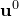To estimate the error in the best-fitting parameter values that we find, we assume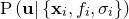to be approximated by an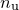-dimensional Gaussian distribution around. Taking a Taylor expansion of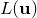about, we can write: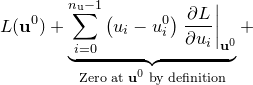(C.4)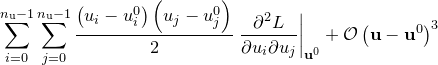Since the logarithm of a Gaussian distribution is a parabola, the quadratic terms in the above expansion encode the Gaussian component of the probability distributionabout.1 We may write the sum of these terms, which we denote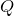, in matrix form: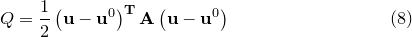(C.5)

where the superscript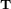represents the transpose of the vector displacement from, andis the Hessian matrix of, given by: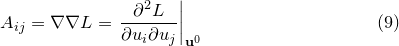(C.6)

This is the Hessian matrix which is output by the fit command. In general, an-dimensional Gaussian distribution such as that given by Equation () yields elliptical contours of equi-probability in parameter space, whose principal axes need not be aligned with our chosen coordinate axes – the variables. The eigenvectors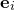ofare the principal axes of these ellipses, and the corresponding eigenvaluesequal, where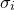is the standard deviation of the probability density function along the direction of these axes.

This can be visualised by imagining that we diagonalise, and expand Equation () in our diagonal basis. The resulting expression foris a sum of square terms; the cross terms vanish in this basis by definition. The equations of the equi-probability contours become the equations of ellipses: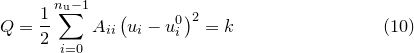(C.7)

whereis some constant. By comparison with the equation for the logarithm of a Gaussian distribution, we can associate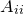with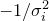in our eigenvector basis.

The problem of evaluating the standard deviations of our variables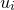is more complicated, however, as we are attempting to evaluate the width of these elliptical equi-probability contours in directions which are, in general, not aligned with their principal axes. To achieve this, we first convert our Hessian matrix into a covariance matrix.

Footnotes

1. The use of this is called Gauss’ Method. Higher order terms in the expansion represent any non-Gaussianity in the probability distribution, which we neglect. See MacKay, D.J.C., Information Theory, Inference and Learning Algorithms, CUP (2003).